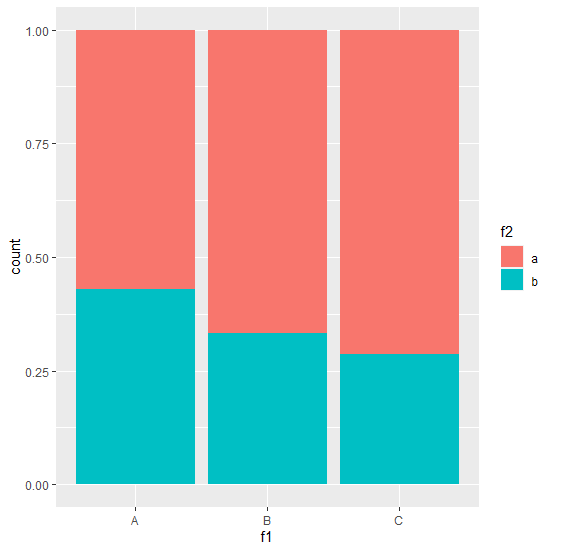# Create stacked bar chart with percentages on Y-axis using ggplot2 in R.

To create stacked bar chart with percentages on Y-axis using ggplot2 in R, we can use fill argument inside geom_bar and put the second categorical variable with position set to fill.

For Example, if we have a data frame called with two categorical columns say C1 and C2 then we can create stacked bar chart with percentages on Y-axis using the below mentioned command −

ggplot(df,aes(C1))+geom_bar(aes(fill=C2),position="fill")

## Example

Following snippet creates a sample data frame −

f1<-sample(LETTERS[1:3],20,replace=TRUE)
f2<-sample(letters[1:2],20,replace=TRUE)
df<-data.frame(f1,f2)
df

The following dataframe is created

  f1 f2
1 B b
2 C a
3 A b
4 A a
5 A a
6 C a
7 B a
8 B a
9 C b
10 B a
11 A a
12 C a
13 A b
14 C b
15 C a
16 B b
17 A b
18 B a
19 A a
20 C a

To load ggplot2 package and create stacked bar chart for columns f1 and f2 with percentages on Y-axis on the above created data frame, add the following code to the above snippet −

f1<-sample(LETTERS[1:3],20,replace=TRUE)
f2<-sample(letters[1:2],20,replace=TRUE)
df<-data.frame(f1,f2)
library(ggplot2)
ggplot(df,aes(f1))+geom_bar(aes(fill=f2),position="fill")

## Output

If you execute all the above given snippets as a single program, it generates the following Output −